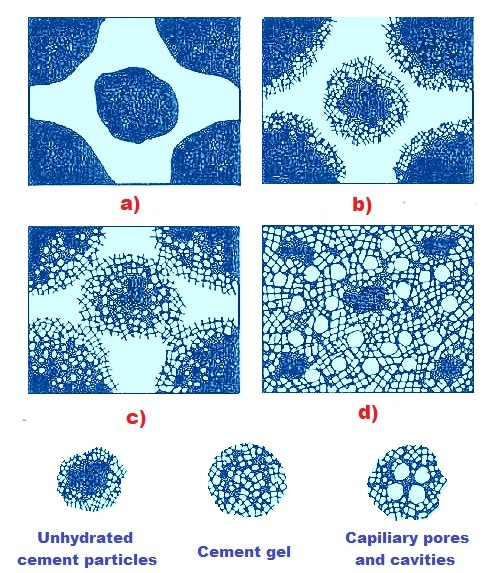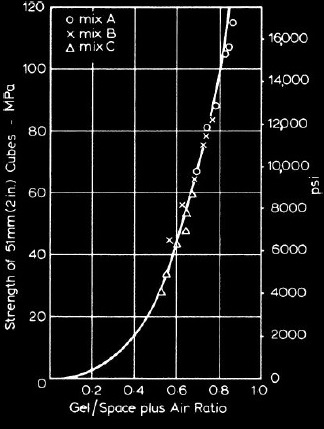# What is gel/space ratio? Its significance in concrete strength prediction

### Gel/space ratio

The ratio of volume occupied by hydrated cement paste to the aggregated volume of capillary pores and hydrated cement paste is known as gel/space ratio. It is denoted by r. Powers (1958) found that compressive strength of concrete is 34000 r3 psi (234 r3 MPa) and interestingly he found no influence of mix proportion of concrete and age of it on strength prediction. To realize the definition and significance of gel/space ratio it is required to discuss about volume of hydration product.

Volume of hydration produts

The total space available to occupy by productsof hydration is the summation of absolute volume of fresh cement and the volume of mixing water. Of these, if small loss of water under the contraction of the cement paste and that due to bleeding is ignored, the water consumed by chemical reaction with C2S and C3S was found to be 21 and 24 percent (very roughly) of the mass of two respective silicates.

If the final reaction of hydrate C4AF is

The respective figures of C3AF and C3A are 37 and 40 percent. Equation (1) is also vary approximately due to our inadequate knowledge of stoichiometry of the hydration products and cannot be ascertained the amount of chemically combined water.Figure-1: Gel formation in cement paste (Paul Bennison)   a) Cement paste just after mixing        b) Initial stiffening-starting reaction after mixing      c) Initial hardening-formation of physical structure      d) Later gradual hardening-infilling pores with gel
Non-evaporable water determined under specific conditions is considered as 23% if anhydrous cement (measured by mass); in case of type II, moderate sulfate resistant cement, this value may be 18%. The specific gravity of hydration products of cement becomes such that the resulting volume is more than absolute volume of anhydrous cement.

The average value of specific gravity of hydration product in saturated structure, inclusive of pores available in the possible densest structure, is 2.16.
Here we are providing a demonstration of calculation of volume change during hydration.

Example 1.0

Mass of cement=100 g
Specific gravity of cement=3.15
That is absolute volume of hydrated cement=100/3.15=31.8 ml
Volume of non-evaporable water= 23ml (23% of mass of cement)
Volume of solid product of hydration of cement=31.8+0.23 X 100 (1-0.254)
=48.9 ml

The cement paste at this condition has characteristic porosity around=28%That is

Where wg=volume of gel water

i.e, wg=48.9X0.28+0.28Xwg
or, wg=19ml

Thus the volume of hydrated cement paste=48.9+19=67.9 ml

The summary of the example 1 can be drawn as in table-1

From table -1 it can be concluded that

Total volume of water in the mix=23+19=42 ml
Water/cement ration= 42/100=0.42 (by mass)
Water/cement ration= 42/31.8=1.32 (by volume)
Actual volume cement+water=31.8+42=73.8 ml

Volume reduction during hydration=73.8-67.9= 5.9 ml
Volume of hydration products for 1 ml of dry cement=67.9/31.8=2.13 ml

Example 1.0 is ideal condition, where hydration is assumed to occur in sealed container where no water movement is allowed whether into or away from the system. It is interesting to note that reduction in volume by value 5.9 stand for empty capillary pores dispersed in the hydrated cement paste.

From the example 1.0, it is found that after hydration of cement, it occupies two times of its actual volume (i.e. 2.13 ml of 1 ml cement). Because all products of hydration are not gel, in the following discussion we assume hydration of 1 unit cement will occupy about 2.06 units (volumetric unit). From the definition of gel/space ratio
Where

C = Mass of cement in mix

Vc = Specific
volume or volume occupied by unit mass of cement

f = Fraction of cement
hydrated

wo=volume of water in the mix

Thus

If specific volume of cement in dry state is considered 0.319 ml/gm above equation becomes

Relation between strength and water/cement ratio
we know a reasonably low water/cement ratio will produce more strength, Too low water cement ratio may reduce workability and may supply insufficient water to hydrate significant fraction of cement incorporated in the mix. A too high water/cement ratio produces a porous structure leaving concrete of inferior quality both strength and durability point of view. But this relationship with water/cement ratio does not based on a well constituted law as water/cement ratio rule cannot be validated by proper qualification.
Strength of concrete at any water/cement ratio depends on other factors like

• Physical and chemical properties of cement
• Degree of hydration of it
• Ambient environment around hydration process like air content in the concrete and temperature.
• Change in effective w/c ratio
• Crack formation due to bleeding
• The cement content in the mix
• Properties of interface between aggregate cement paste

### Relation between strength and gel-space ratio

As water/cement ratio is not sole factor to control strength of concrete, it is more accurate to relate strength with the distribution and concentration of solid materials produced from hydration of cement in the available space for these materials. Power (1946) has first determined the relation between development of strength and gel/space ratio of mortar has shown in following figure.Figure-2: Relation between development of strength and gel/space ratio of mortar

From the figure-2, it is noticed that strength has approximate proportionality with cube of gel/space ratio and he figured out that 234 Mpa was the inherent strength of gel for that type of cement and specimen examined at that time. For usual Portland cement we used in our construction works, the numerical vales were found more or less same; some exceptions are observed for cements having higher C3A content as they yield lower strength at a particular gel/space ratio.

### Correction for specific gravity of adsorbed water

In discussing this topic, some question need to be answered, these are:

### What is disjoining pressure?

An investigations applying nuclear magnetic resonance showed that gel water required same energy to make bond as that remain in interlayer water of some expansive clays; thus is can be said that gel water is a form of interlayer water. The non-evaporable water calculated in example 1.0 comprises approximately all chemically bonded water and some water that is not chemically bonded. Such water remains in lower vapor pressure than the pressure of the surrounding atmosphere; thus quantity of this water may vary depending on ambient vapor pressure.
The energy of binding water in cement paste depends on the manner of holding water in it; as an example to establish bond of 1gm non-evaporable water 850 calories (1670J) are used and energy required to crystallize 1 gm water of Ca(OH)

2 is 850 cal (3560J).Similarly the specific gravity of water taken as 1.2 in case of non-evaporable water and this value is 1.1 for gel water and 1.0 in case of free water as usual.

Don’t be confused about the increase in density due to compression at low surface concentrations; the actual cause is the ordering or orientation of the molecules of water in the adsorbed phase under the action of surface forces which results a pressure called disjoining pressure.

This pressure is required to maintain the film of adsorbed molecules of water against external influence.

Thus the calculation of gel/space ratio requires a little modification to encounter revise specific gravity of water. Therefore the actual situation is that volume of pores will be larger to some degree as we assumed.

Correction for air content

If the volume of air exists in the cement paste is A, the equation (2) the ratio of W0/C will be replaced by
The expression for compressive strength will be
Feret (1896) suggest an expression for strength of concreteFigure-3: Relationship between compressive  strength and gel/space ratio of mortar with  correction for entrapped air.

Where C, w and a represents absolute volumetric proportions for cement, water and air respectively

K=constant
Power’s expression for strength (3) is somewhat similar to that provided by Feret (4); the exception in power expression is he took account for values that are proportional to amount of hydrated cement replacing total volume of cement. As cement hydrates with time, the relationship between strength and gel/space ratio is independent of age of concrete. Figure-3 shows relationship between compressive strength and gel/space ratio of mortar with correction for entrapped air.

### Simplification of relationship

The expression for relationship between strength and gel/space ratio can be used in several ways. For simplification and convenient to use consider
• Wn α volume of gel, when wn=non-evaporable water in the mix.
• W
0 represents available space for the gel

The expression for strength can be written as follows when f
c in psi fc>2000 psi

Equation (5) shows a linear relationship.
An alternative expression can be written in terms of surface area of gel v
m.

Figure-4 was established by power (1947) based on actual data for cement having low C3A content.Figure-4: Relationship between the strength of cement paste and the ratio of surface area of gel Vm to the volume of mixing water w0

### Effect of temperature on gel/space ratio

We know a higher temperature at the time of placing of concrete and maintaining or allowing during setting, definitely increase strength at very early stage; followed by often adverse effect on strength at and after 7 days. This is due to rapid initial reaction that occur during hydration leading to formation of hydration product of poorer quality; a poor physical structure having more pores.Thus a significant portion of pores will always leave unfilled. According to gel/space ratio rule, the gel/space ratio will be lower in this case as compared to concrete that is hydrated slowly. The latter one will have less pores, thus resulting higher gel/space ratio.According to Verbeck and Helmuth, the explanation of low gel/space ratio due to high early temperature and subsequent adverse effects on later strength of concrete, is higher rate of hydration at initial stage under higher temperature inhibit successive hydration. . This is due to an irregular distribution of hydration products in the paste. Diffusion away of the hydration product, in this case, takes inadequate time from cement particles under initial higher temperature and cannot produce uniform precipitation into interstices of paste.

Thus high density of hydration product is produced in vicinity of particles still waiting for hydration reaction or in state of some degree of hydration and inhibit subsequent hydration. As a result gel/space ratio will be less in the interstices and adversely affects strength of concrete in the long run. Thus the local weakness in the concrete section will permit to progress cracks and results a lower strength of it as a whole. With the help backscattered electron imaging it has been confirmed that porous C-S-H exist in between hydrated cement particles.

### Influence of chemical composition of cement

As per chemical composition, only gypsum content of cement is here prime concern. For a given cement, the gypsum content defines shrinkage and setting time of cement; but the gypsum content that is satisfactory in respect of shrinkage is found not adequate for establishing desired setting time. Gypsum is added to cement clinker during manufacturing process of cement and then final grinding of clinker is done. As discussed in above section about temperature, the initial structure produced during setting determines structure of cement paste at upcoming stages of hydration. Thus gel/space ratio is dependent on optimum gypsum content to facilitate hydration of as much as cement particles to gain strength and behavior of concrete under shrinkage and creep are also affected.